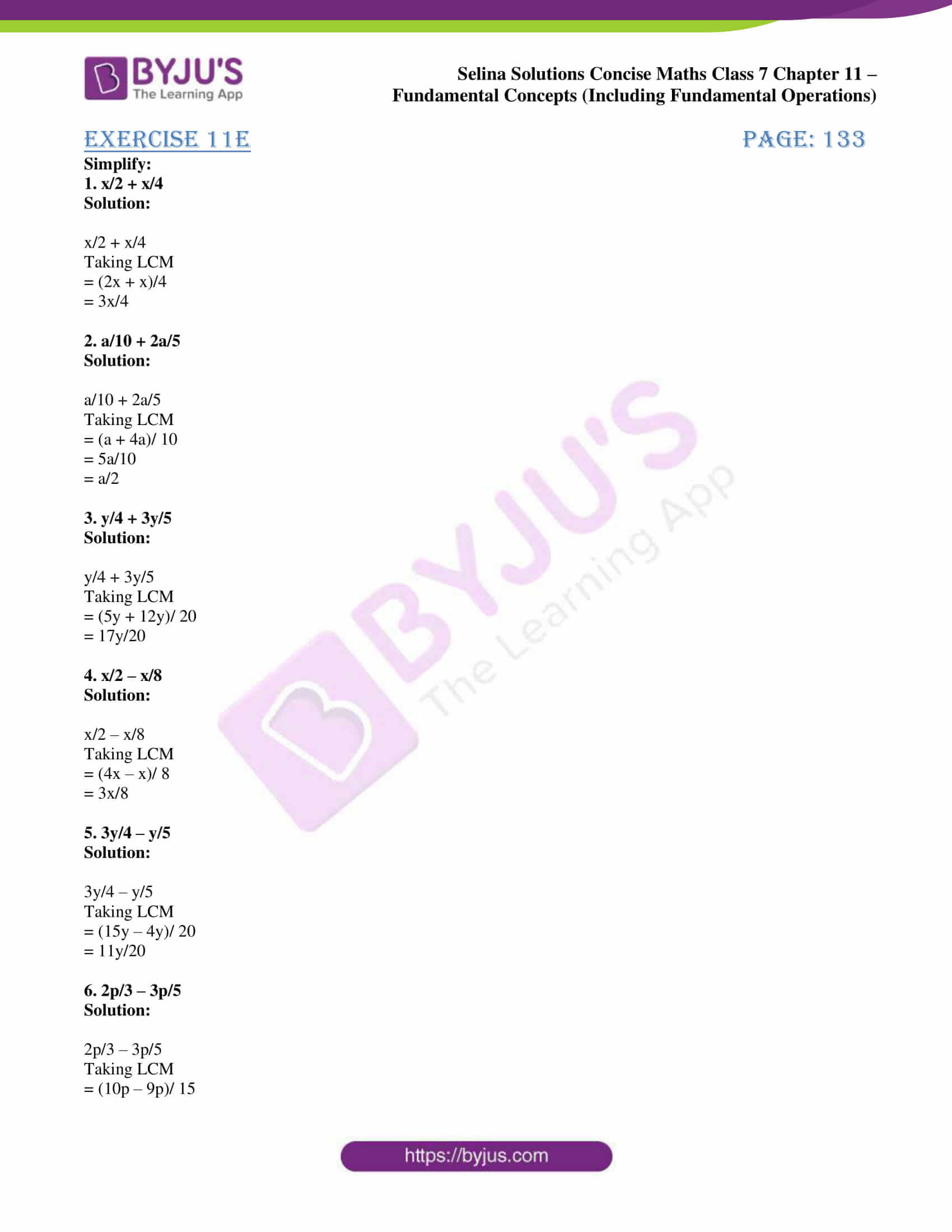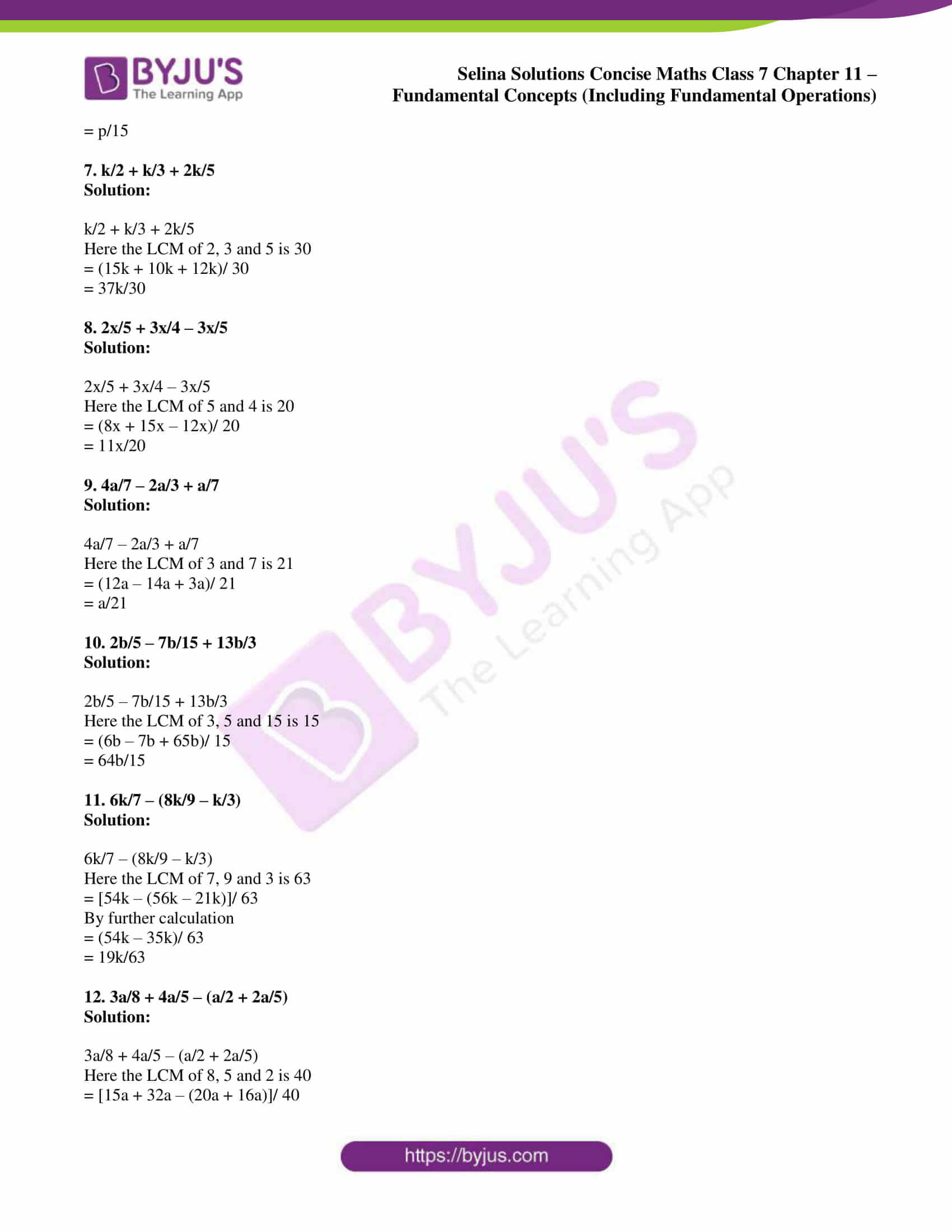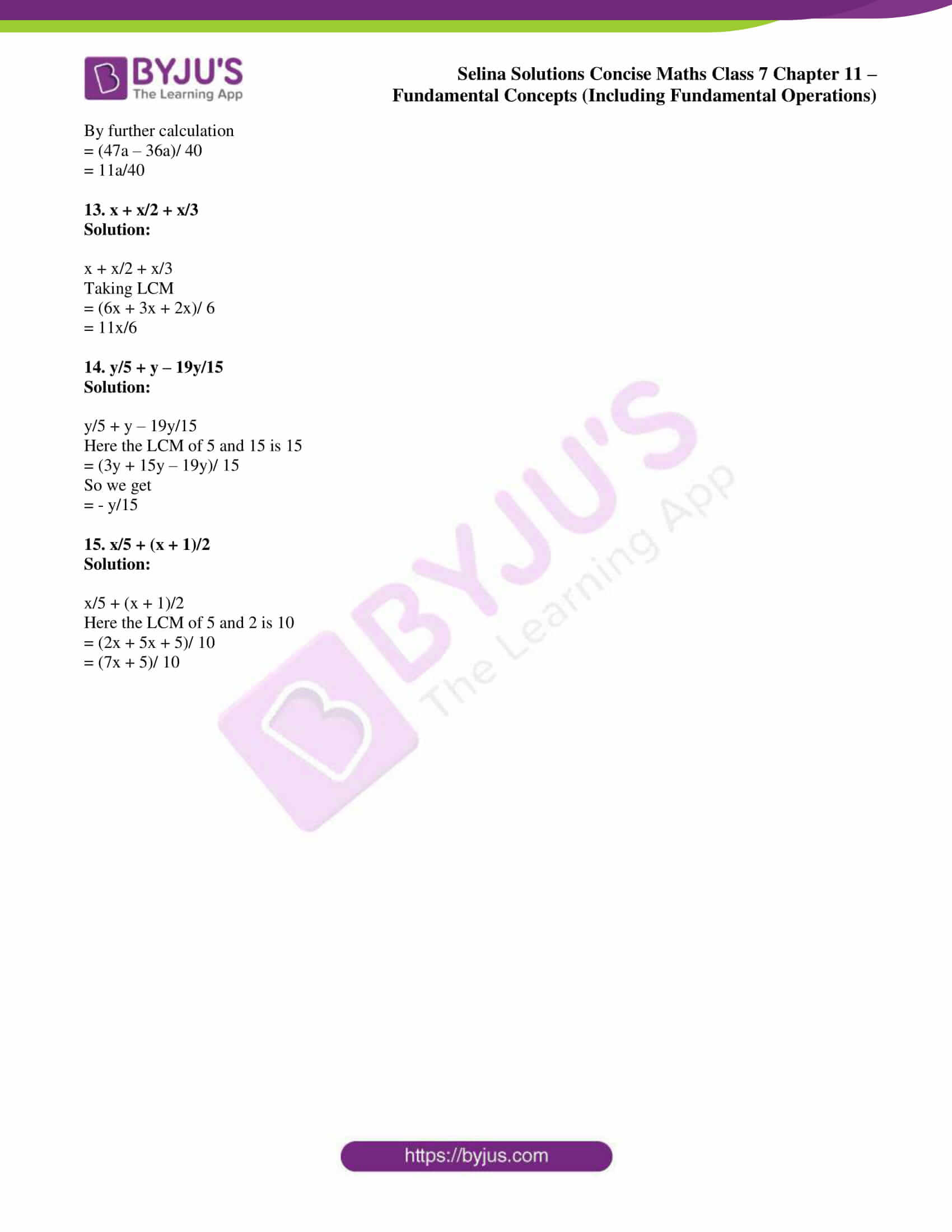# Selina Solutions Concise Maths Class 7 Chapter 11: Fundamental Concepts (Including Fundamental Operations) Exercise 11E

Selina Solutions Concise Maths Class 7 Chapter 11 Fundamental Concepts (Including Fundamental Operations) Exercise 11E explains the concept of combining algebraic expressions with integral denominators. The solutions contain solved examples, which help students analyze the type of problems that would appear in the annual exam. The PDF format of these solutions can be used by the students to cross-check their answers and solve doubts by themselves. Students can use Selina Solutions Concise Maths Class 7 Chapter 11 Fundamental Concepts (Including Fundamental Operations) Exercise 11E free PDF, from the links which are given below.

## Selina Solutions Concise Maths Class 7 Chapter 11: Fundamental Concepts (Including Fundamental Operations) Exercise 11E Download PDF### Access other exercises of Selina Solutions Concise Maths Class 7 Chapter 11: Fundamental Concepts (Including Fundamental Operations)

Exercise 11A Solutions

Exercise 11B Solutions

Exercise 11C Solutions

Exercise 11D Solutions

Exercise 11F Solutions

### Access Selina Solutions Concise Maths Class 7 Chapter 11: Fundamental Concepts (Including Fundamental Operations) Exercise 11E

#### Exercise 11E page: 133

Simplify:

1. x/2 + x/4

Solution:

x/2 + x/4

Taking LCM

= (2x + x)/4

= 3x/4

2. a/10 + 2a/5

Solution:

a/10 + 2a/5

Taking LCM

= (a + 4a)/ 10

= 5a/10

= a/2

3. y/4 + 3y/5

Solution:

y/4 + 3y/5

Taking LCM

= (5y + 12y)/ 20

= 17y/20

4. x/2 – x/8

Solution:

x/2 – x/8

Taking LCM

= (4x – x)/ 8

= 3x/8

5. 3y/4 – y/5

Solution:

3y/4 – y/5

Taking LCM

= (15y – 4y)/ 20

= 11y/20

6. 2p/3 – 3p/5

Solution:

2p/3 – 3p/5

Taking LCM

= (10p – 9p)/ 15

= p/15

7. k/2 + k/3 + 2k/5

Solution:

k/2 + k/3 + 2k/5

Here the LCM of 2, 3 and 5 is 30

= (15k + 10k + 12k)/ 30

= 37k/30

8. 2x/5 + 3x/4 – 3x/5

Solution:

2x/5 + 3x/4 – 3x/5

Here the LCM of 5 and 4 is 20

= (8x + 15x – 12x)/ 20

= 11x/20

9. 4a/7 – 2a/3 + a/7

Solution:

4a/7 – 2a/3 + a/7

Here the LCM of 3 and 7 is 21

= (12a – 14a + 3a)/ 21

= a/21

10. 2b/5 – 7b/15 + 13b/3

Solution:

2b/5 – 7b/15 + 13b/3

Here the LCM of 3, 5 and 15 is 15

= (6b – 7b + 65b)/ 15

= 64b/15

11. 6k/7 – (8k/9 – k/3)

Solution:

6k/7 – (8k/9 – k/3)

Here the LCM of 7, 9 and 3 is 63

= [54k – (56k – 21k)]/ 63

By further calculation

= (54k – 35k)/ 63

= 19k/63

12. 3a/8 + 4a/5 – (a/2 + 2a/5)

Solution:

3a/8 + 4a/5 – (a/2 + 2a/5)

Here the LCM of 8, 5 and 2 is 40

= [15a + 32a – (20a + 16a)]/ 40

By further calculation

= (47a – 36a)/ 40

= 11a/40

13. x + x/2 + x/3

Solution:

x + x/2 + x/3

Taking LCM

= (6x + 3x + 2x)/ 6

= 11x/6

14. y/5 + y – 19y/15

Solution:

y/5 + y – 19y/15

Here the LCM of 5 and 15 is 15

= (3y + 15y – 19y)/ 15

So we get

= – y/15

15. x/5 + (x + 1)/2

Solution:

x/5 + (x + 1)/2

Here the LCM of 5 and 2 is 10

= (2x + 5x + 5)/ 10

= (7x + 5)/ 10